The Continuous Ranked Probability Score, known as CRPS, is a score to measure how a proposed distribution approximates the data, without knowledge about the true distributions of the data.

## Definition

CRPS is defined as1

$$CRPS(P, x_a) = \int_{-\infty}^\infty \lVert P(x) - H(x - x_a) \rVert_2 dx,$$

where

• $x_a$ is the true value of $x$,
• P(x) is our proposed cumulative distribution for $x$,
• $H(x)$ is the Heaviside step function

$$H(x) = \begin{cases} 1, &\qquad x=0\\ 0, &\qquad x\leq 0\\ \end{cases}$$

• $\lVert \cdot \rVert_2$ is the L2 norm.

## Explain it

The formula looks abstract on first sight, but it becomes crystal clear once we understand it.

Note that the distributions that corresponds to a Heaviside CDF is the delta function $\delta(x-x_a)$. What this score is calculating is the difference between our distribution and a delta function. If we have a model that minimizes CRPS, then we are looking for a distribution that is close to the delta function $\delta(x-x_a)$. In other words, we want our distribution to be large around $x_a$.

To illustrate what the integrand $\lVert P(x) - H(x - x_a) \rVert_2$ means, we consider several scenarios.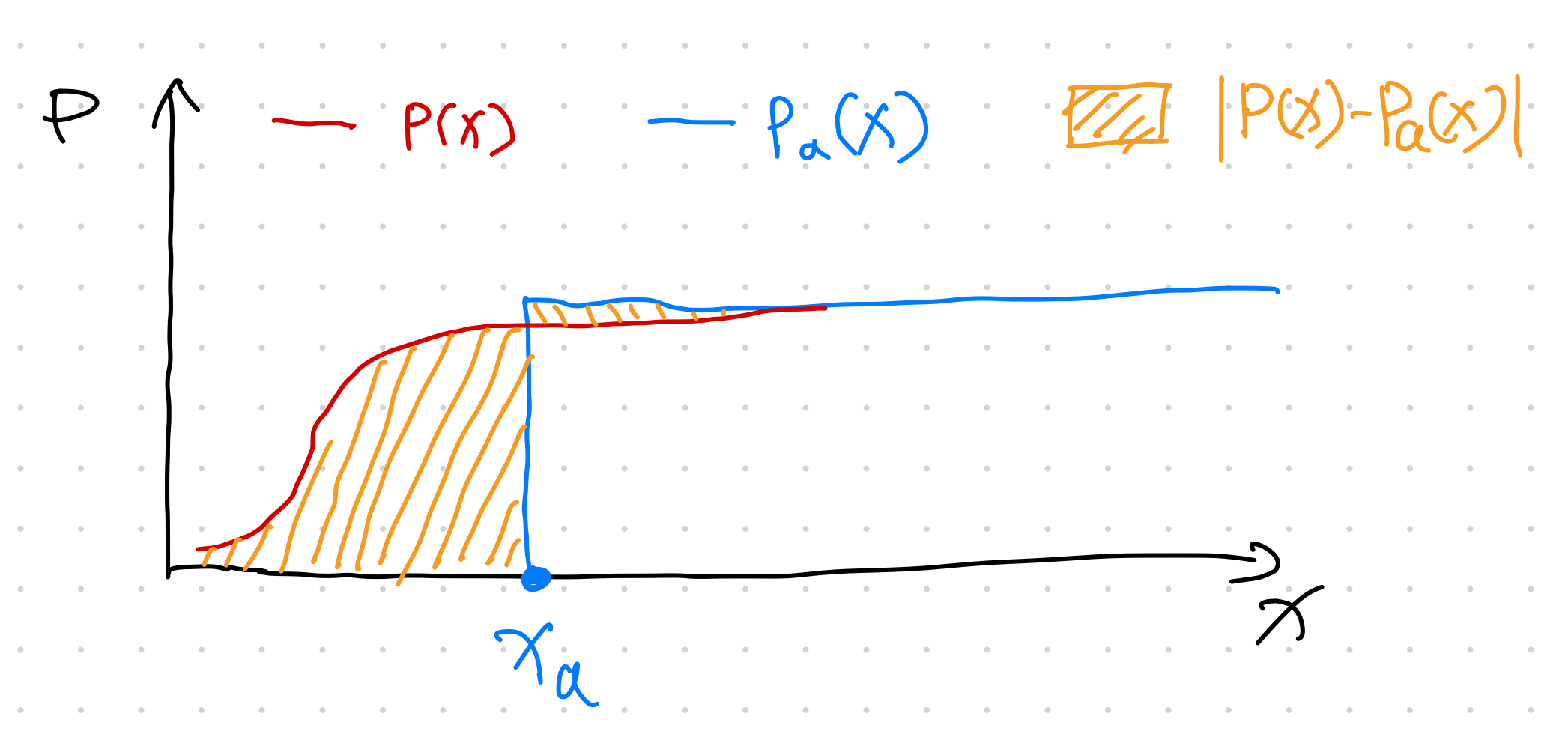When the proposed CDF $P(x)$ is reaching 1 faster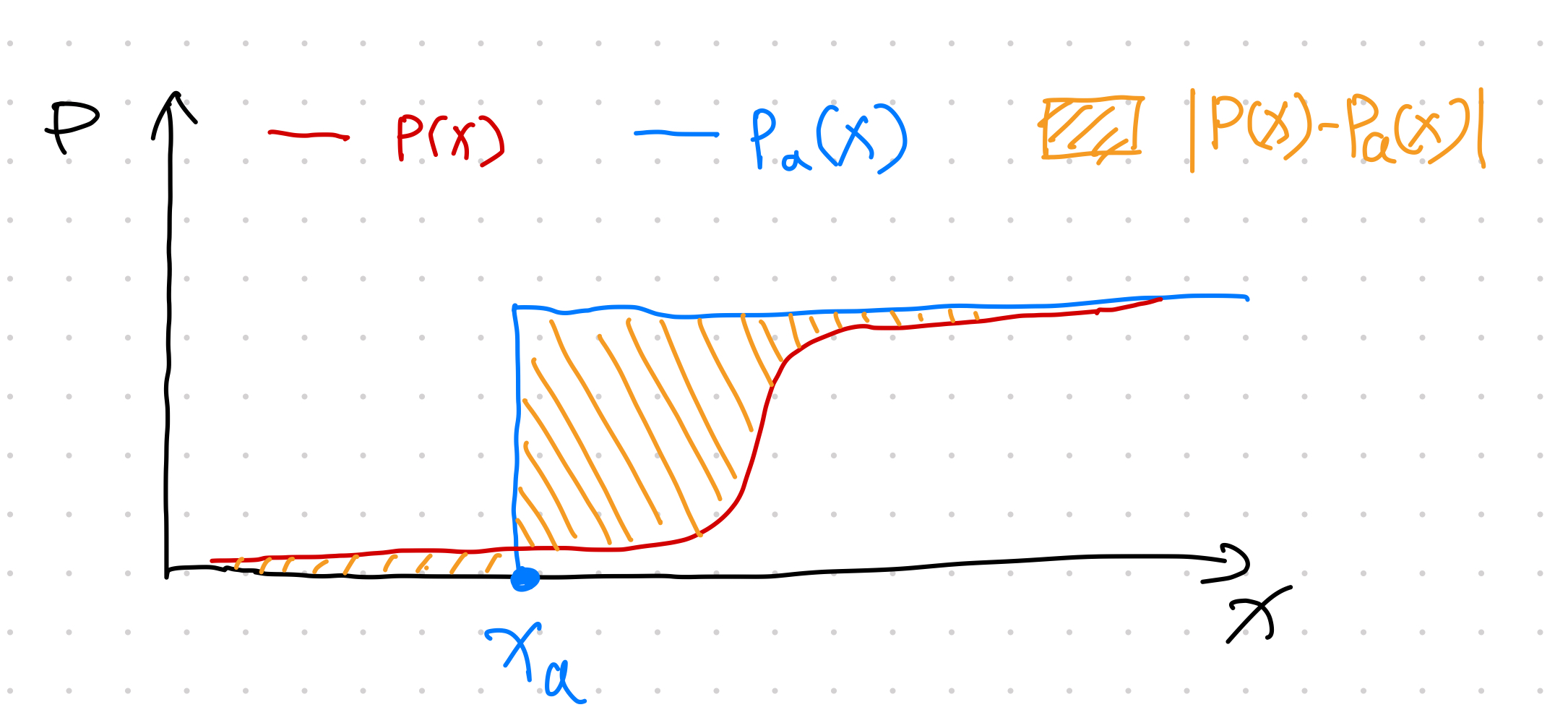When the proposed CDF $P(x)$ is reaching 1 slower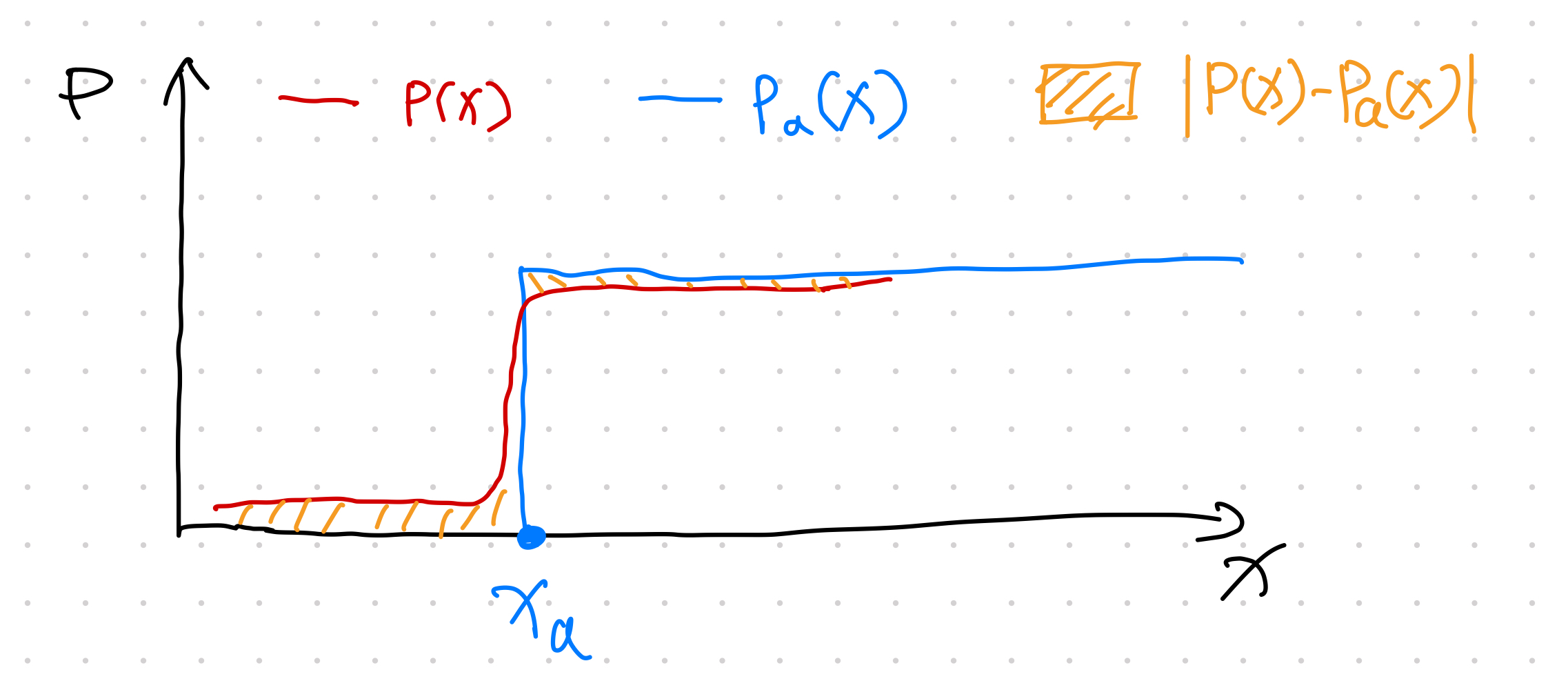When the proposed CDF $P(x)$ is close to the Heaviside function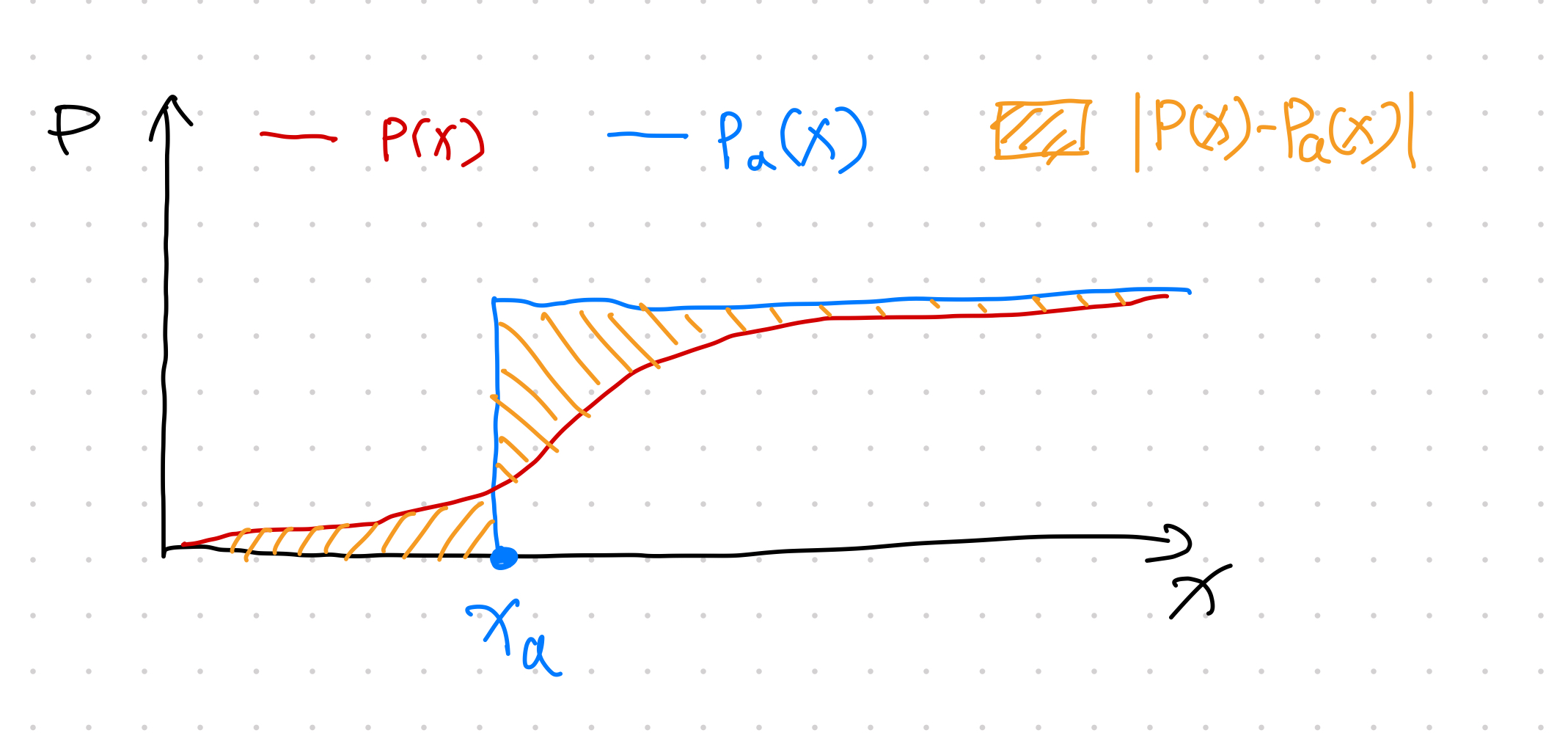When the proposed CDF $P(x)$ is dispersed around $x_a$

The shade areas determines the integrand of the integral in CRPS. The only way to get a small score is to choose a distribution that is focused around $x_a$.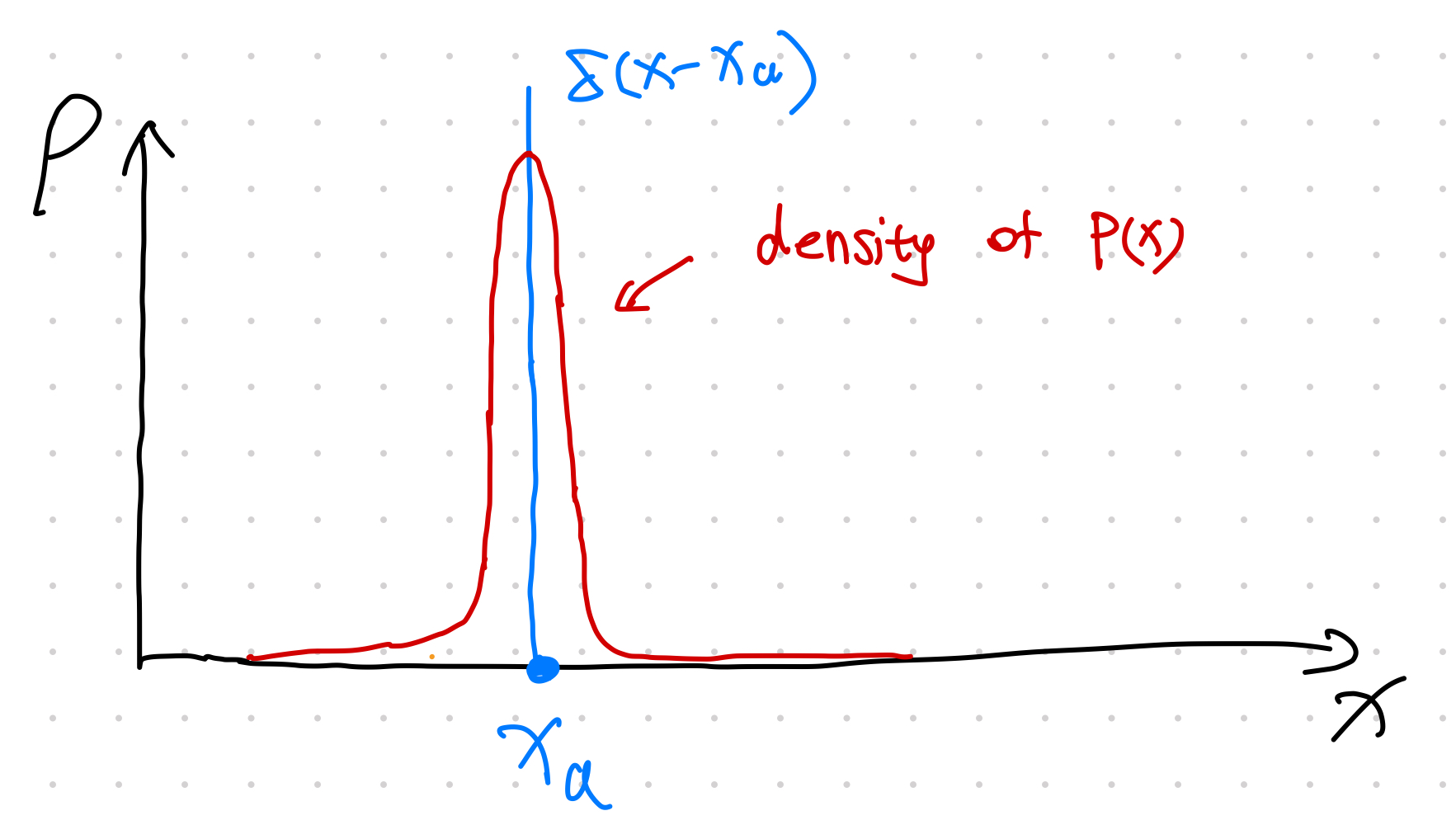densities of $P(x)$ and $H(x-x_a)$

## Compared to f-Divergence

Compared to or more generally , CRPS is comparing our proposed CDF to the Heaviside CDF.

f-Divergence
The f-divergence is defined as1 $$\operatorname{D}_f = \int f\left(\frac{p}{q}\right) q\mathrm d\mu,$$ where $p$ and $q$ are two densities and $\mu$ is a reference distribution. Requirements on the generating function The generating function $f$ is required to be convex, and $f(1) =0$. For $f(x) = x \log x$ with $x=p/q$, f-divergence is reduced to the KL divergence  \begin{align} &\int f\left(\frac{p}{q}\right) q\mathrm d\mu \\ =& \int \frac{p}{q} \log \left( \frac{p}{q} \right) \mathrm …
KL Divergence
Kullback–Leibler divergence indicates the differences between two distributions

## Compared to Likelihood

Gebetsberger et al found that CRPS is more robust but produces similar results if we have found a good assumption about the data distribution2.

## Applications

One quite interesting application of the CRPS is to write down the loss for an the quantile function3.

Planted: by ;

L Ma (2022). 'Continuous Ranked Probability Score - CRPS', Datumorphism, 03 April. Available at: https://datumorphism.leima.is/cards/time-series/crps/.